Next: Kepler's Second Law Up: Planetary Motion Previous: Polar Coordinates

# Conic Sections

The ellipse, the parabola, and the hyperbola are collectively known as conic sections, since these three types of curve can be obtained by taking various different plane sections of a right cone. It turns out that the possible solutions of Equations (228) and (229) are all conic sections. It is, therefore, appropriate for us to briefly review these curves.

An ellipse, centered on the origin, of major radius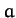and minor radius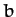, which are aligned along the- and-axes, respectively (see Figure 14), satisfies the following well-known equation: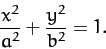(230)

Likewise, a parabola which is aligned along the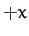-axis, and passes through the origin (see Figure 15), satisfies: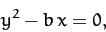(231)

where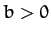.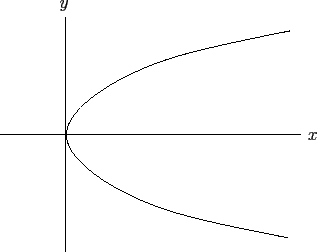Finally, a hyperbola which is aligned along the-axis, and whose asymptotes intersect at the origin (see Figure 16), satisfies: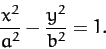(232)

Here,is the distance of closest approach to the origin. The asymptotes subtend an angle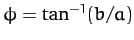with the-axis.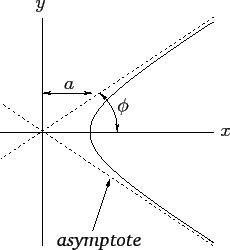It is not clear, at this stage, what the ellipse, the parabola, and the hyperbola have in common (other than being conic sections). Well, it turns out that what these three curves have in common is that they can all be represented as the locus of a movable point whose distance from a fixed point is in a constant ratio to its perpendicular distance to some fixed straight-line. Let the fixed point (which is termed the focus of the ellipse/parabola/hyperbola) lie at the origin, and let the fixed line correspond to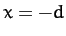(with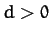). Thus, the distance of a general point (,) (which lies to the right of the line) from the origin is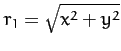, whereas the perpendicular distance of the point from the lineis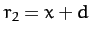--see Figure 17. In polar coordinates,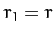and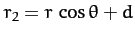. Hence, the locus of a point for which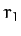and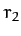are in a fixed ratio satisfies the following equation: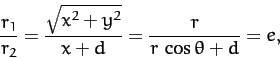(233)

where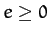is a constant. When expressed in terms of polar coordinates, the above equation can be rearranged to give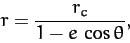(234)

where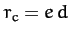.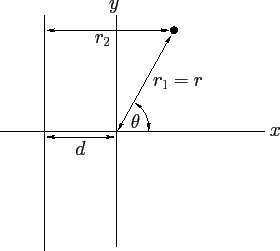When written in terms of Cartesian coordinates, (233) can be rearranged to give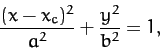(235)

for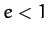. Here,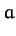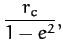(236)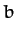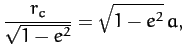(237)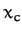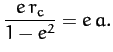(238)

Equation (235) can be recognized as the equation of an ellipse whose center lies at (,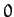), and whose major and minor radii,and, are aligned along the- and-axes, respectively [cf., Equation (230)].

When again written in terms of Cartesian coordinates, Equation (233) can be rearranged to give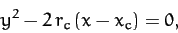(239)

for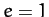. Here,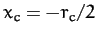. This is the equation of a parabola which passes through the point (,), and which is aligned along the-direction [cf., Equation (231)].

Finally, when written in terms of Cartesian coordinates, Equation (233) can be rearranged to give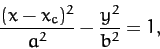(240)

for. Here,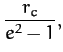(241)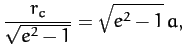(242)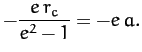(243)

Equation (240) can be recognized as the equation of a hyperbola whose asymptotes intersect at (,), and which is aligned along the-direction. The asymptotes subtend an angle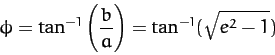(244)

with the-axis [cf., Equation (232)].

In conclusion, Equation (234) is the polar equation of a general conic section which is confocal with the origin. For, the conic section is an ellipse. For, the conic section is a parabola. Finally, for, the conic section is a hyperbola.Next: Kepler's Second Law Up: Planetary Motion Previous: Polar Coordinates
Richard Fitzpatrick 2011-03-31